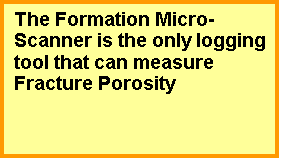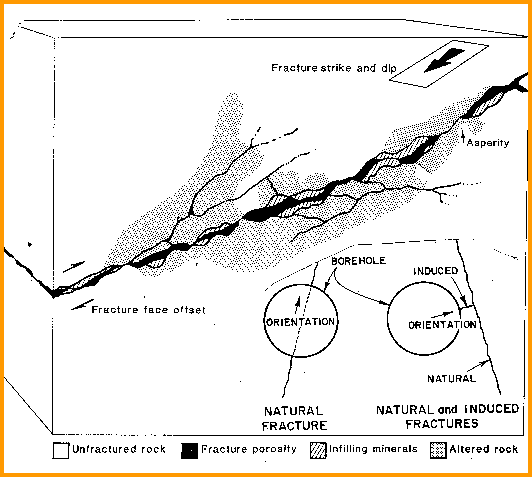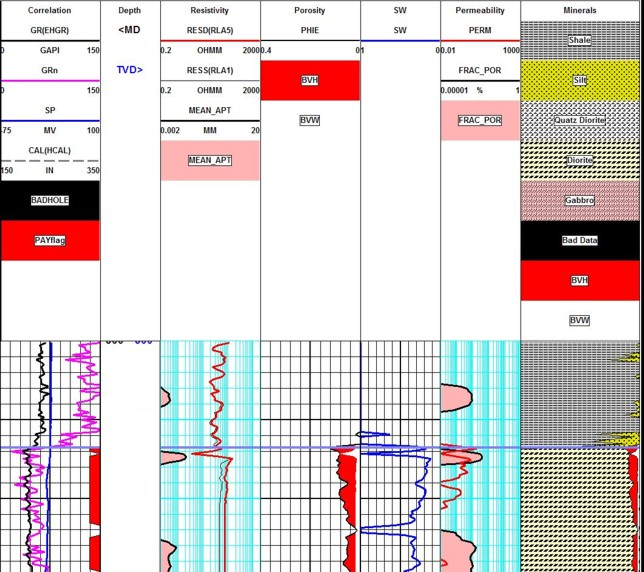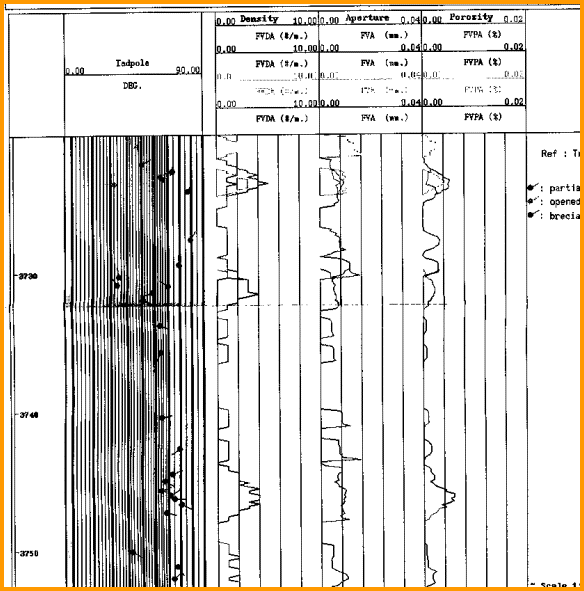Definition of FractureS
A fracture is sometimes called a joint and, at the surface, are expressed as cracks or fissures in the rocks. The orientation of the fracture can be anywhere from horizontal to vertical. The rough surface separates the two faces, giving rise to fracture porosity. The surfaces touch at points called asperities. Altered rock surrounds each surface and may contain solution porosity. Infilling minerals may cover part or all of each surface . Minerals may fill the entire fracture, converting an open fracture to a healed or sealed fracture.

Fracture porosity is usually very small. Values between 0.0001 and 0.001 of rock volume are typical (0.01% to 0.1%). Fracture-related porosity, such as solution porosity in granite or carbonate reservoirs, may attain much larger values, but the porosity in the actual fracture is still very small.Fracture porosity is found accurately only by processing the formation micro-scanner curves for fracture aperture and fracture frequency (fracture intensity). Reservoir matrix porosity and permeability, including that attributed to fracture related (solution) porosity, can be found by normal porosity calculation methods.

Reservoir simulation software that accounts for the fracture system is often termed a “dual porosity” model. While this is strictly true, it would be better to think of them as “dual permeability” models, since the fracture permeability fed by the matrix or reservoir permeability is far more important than the relative storage capacity of the fractures and matrix porosity. A reservoir with only fracture porosity is quickly depleted; a decent reservoir in the matrix rock feeding into fractures will last much longer.Fracture Porosity Definitions, showing fracture porosity (black) and fracture related porosity (black dots),

Fractures are caused by stress in the formation, which in turn usually derives from tectonic forces such as folds and faults. These are termed natural fractures, as opposed to induced fractures. Induced fractures are created by drilling stress or by purposely fracturing a reservoir by hydraulic pressure from surface equipment. Both kinds of fractures are economically important. Induced fractures may connect the wellbore to natural fractures that would otherwise not contribute to flow capacity.Fracture POROSITY And PERMEABILITY from APERTURE DATA
Quantitative prediction of porosity and permeability of fractures relies on the fracture aperture, measured in millimeters, from resistivity micro-scanner or observation of cores or core images.
1. PHIfrac = 0.001 * Wf * Df * KF1

The fracture permeability equations are attributed to Dr Zoltan Barlai:
2: Kfrac = 833 * 10^11 * PHIfrac^3 / (Df^2 * KF1^2)
3: Kfrac = 833 * 10^5 * PHIfrac * Wf^2
4: Kfrac = 833 * 10^2 * Wf^3 * Df * KF1

Where:
KF1 = number of main fracture directions
= 1 for sub-horizontal or sub-vertical
= 2 for orthogonal sub-vertical
= 3 for chaotic or brecciated
PHIfrac = fracture porosity (fractional)
Df = fracture frequency (fractures per meter)
Wf = fracture aperture (millimeters)
Kfrac = fracture permeability (millidarcies)

Note: Equations 2, 3, and 4 give identical results.NUMERICAL EXAMPLE
Df = 1 fracture per meter
Wf = 1.0 millimeters
PHIfrac = 0.001 * 1 * 1 = 0.001 fractional (0.1%)
Kfrac = 833 * 100 * 1^3 * 1 * 1 = 83300 millidarcies

Df = 10 fractures per meter
Wf = 0.1 millimeters
PHIfrac = 0.001 * 10 * 0.1 = 0.001 fractional (0.1%)
Kfrac = 833 * 100 * 0.1^3 * 10 * 1 = 833 millidarcies

These examples represent well fractured reservoirs. You can see that the volume of hydrocarbon is very small but the permeability is very high.

SPR-29 META/LOG FRACTURE POROSITY and PERMEABILITY CALCULATOR
Calculate fracture porosity and fracture permeability from fracture aperture - Zoltan Barlai modelsCalculating Fracture APERTURE FROM IMAGE LOG DATA
There are two methods that can be used to determine fracture aperture from image logs. One is to use image processing techniques to "count pixels". By scanning an image and counting the darkest black pixels, and a little judicious use of geometry, the volume of the fractures seen in the image can be estimated.  The method is very sensitive to the trigger level used to descriminate the fracture from surrounding borehole roughness or breakouts. Overestimation is a common outcome. The approach is cheap, easy, and often wrong.Conventional petrophysical analysis with fracture aperture (Track 2 - shaded pink) and fracture porosity (Track 4- shaded oink) both derived from image analysis methods. Note the low resistivity spike on the fracture in the center of the log section, caused by mud filtrate invasion into the fracture.

A more accurate approach is based on finite element analysis of the resistivity image data. as described in  "Fracture Apertures from Electrical Borehole Scans", S. M. Luthi and P. Souhaite, Geophysics, Vo1.55, No.7, July, 1990, pp.821-833. The math is beyond me and beyond the scope of this article. The method is available from some service companies. An example of a fracture aperture log from a program called Frac-View is shown below.Fracture frequency, aperture, and porosity log in a fractured granite reservoir derived from a resistivity image log. The most accurate method is based on the measured resistivity curves on the image log. The pixel count method is much less accurate because of borehole erosion and breakouts.

Page Views ---- Since 01 Jan 2015
Copyright 2023 by Accessible Petrophysics Ltd.
CPH Logo, "CPH", "CPH Gold Member", "CPH Platinum Member", "Crain's Rules", "Meta/Log", "Computer-Ready-Math", "Petro/Fusion Scripts" are Trademarks of the Author